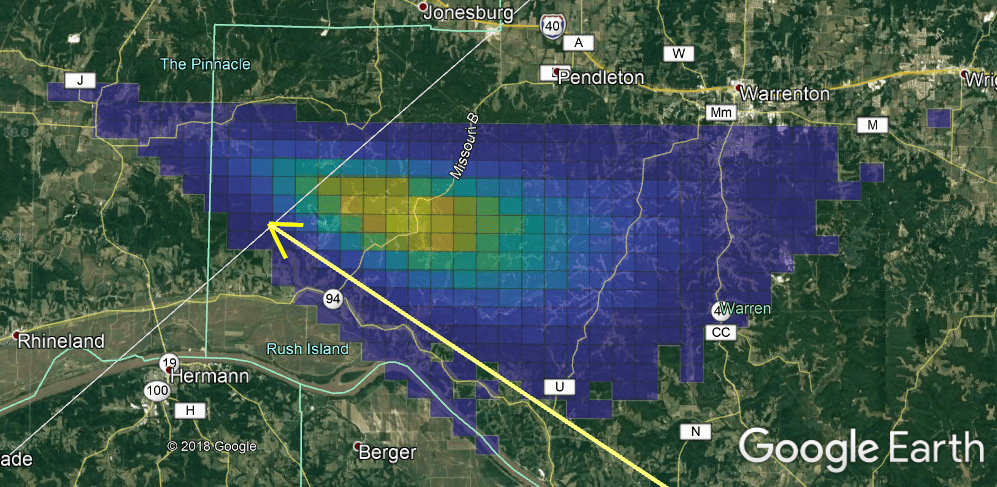September 29, 2023

# Monte Carlo Simulation MethodsI received a question about how I calculate trajectory and I think everyone might be interested in the answer, so I’ll provide it here: My meteor simulation program, which I have named StrewnLAB, takes into account all of the trajectory inputs from the attached spreadsheet, which provides both nominal values and assumed error/variation for each measurement.  You will find the recent event in Germany on row 9, labelled “Flensburg”.  For CNEOS data, I assume the error in latitude and longitude to be 0.05 degrees, because it is provided with a precision of only one decimal place (54.5 N, 9.2 E).  I also have reason to believe that the accuracy may be worse than that, because Chelyabinsk seems to off by more than the expected amount, so I also took the liberty of adjusting the strewn field location with camera data in the latest bulletin, the attached version 2.
So, when the simulation runs, it takes a Monte Carlo approach, generating many possible scenarios using random inputs.  This means it calculates a random number for every input parameter (lat, long, speed, etc), based on the nominal values and the assumed error for that input.  It also uses different distributions (purely random, lognormal, normal, etc), depending on what we know about the data.  For example, I know that the latitude and longitude data is simply rounded to one decimal place, so the distribution is purely random.  However, I know from published works that the distribution of meteorite mass is approximately a lognormal distribution, so the fragmentation model uses that to fragment a random mass from the main mass, at random times during flight.  Another example is the weather data, which is interpolated from multiple radiosonde measurements to the most likely profile at the time and location of the event.  The accuracy of the weather data prediction depends on the stability of the local atmosphere, so I attempt to include that variation by calculating standard deviation of the measurement at each altitude and define the error to be 1.5 sigma.  I consider the interpolated value to be a good average, so the each Monte Carlo scenario is generated using a skew normal distribution of the weather data (I actually clip the values to 1.5 sigma, because the number of scenarios is relatively small and I don’t want an unlikely high sigma number to skew my results.)
In the final result , the probability color scale is based on how many modeled fragments landed in that square, out of all the scenarios.  It is important to note that the actual strewn field is much smaller than the generated strewn field and likely a different shape, because the final result contains all the possible scenario strewn fields “overlapped,” and the probability is based on how those fields overlap.  It is also important to know that the color scale is not comparable between simulations and/or events, because there is no limit to the number of scenarios I can run and the fragmentation is also random.  I typically just let the simulation run overnight and post the results in the morning, which generates anywhere from 20,000 to 80,000 fragments and maybe ~25 scenarios.   I have some ideas on how to improve the simulation speed and thereby improve the “variation coverage” and repeatability, but it is not a big problem at the moment and the solution will take some time to implement into my algorithm.

## 2 thoughts on “Monte Carlo Simulation Methods”

1.Nathan Luetjen says:

Are these objects still moving forward or dropping straight down under the radar bleeps

1.Jim Goodall says:

Sorry for the delayed reply. The answer depends on the altitude of the radar blip and the mass of the material, but for the most part, the fragments are dropping nearly straight down by the time they are detected by radar. If there are large fragments detected high in the atmosphere, they would still have forward momentum, for example. Or, if the fragments are small, they could be significantly be impacted by wind after they are detected. Using the StrewnLAB software, we can predict the fall zone from the radar blips, using the wind profile. Very large masses (>10kg) can even carry forward momentum all the way to the ground, but they usually impact at only a few degrees from vertical (this is confirmed by the angle of impact holes through structures).